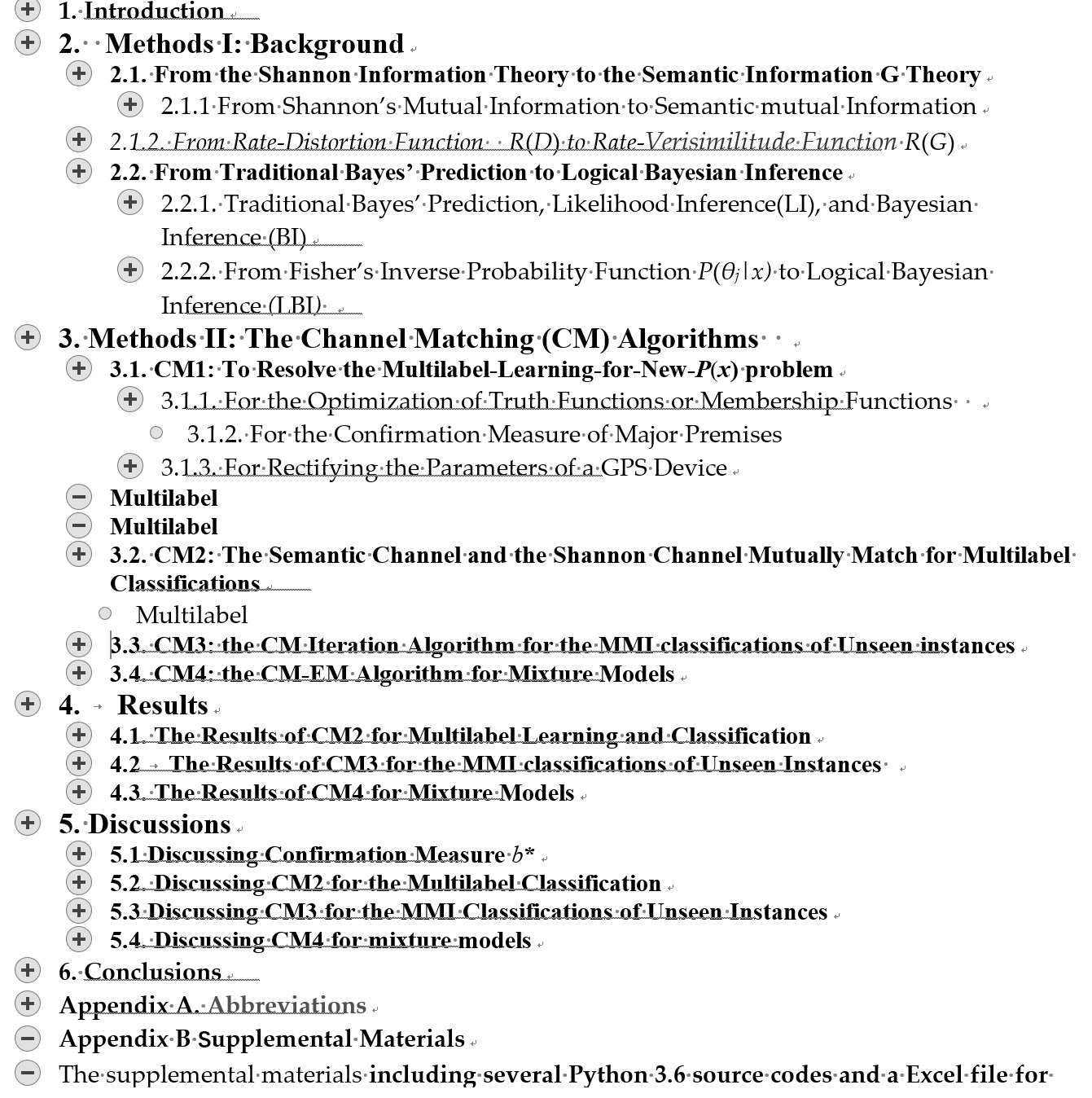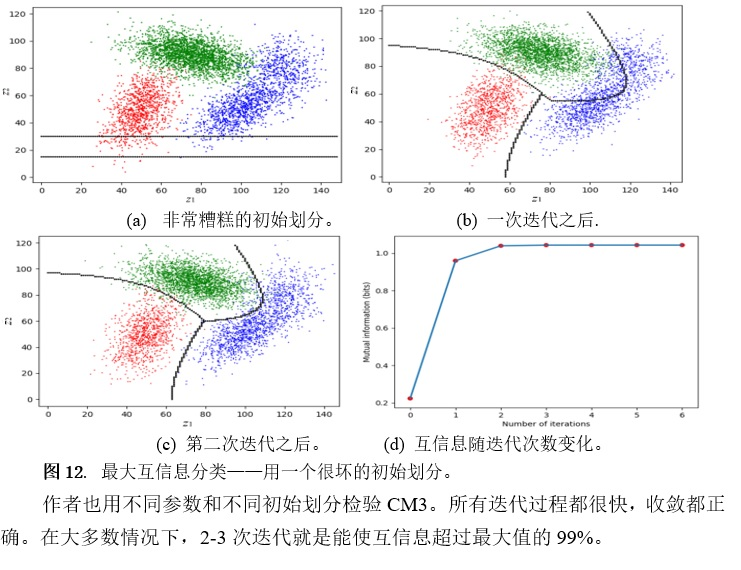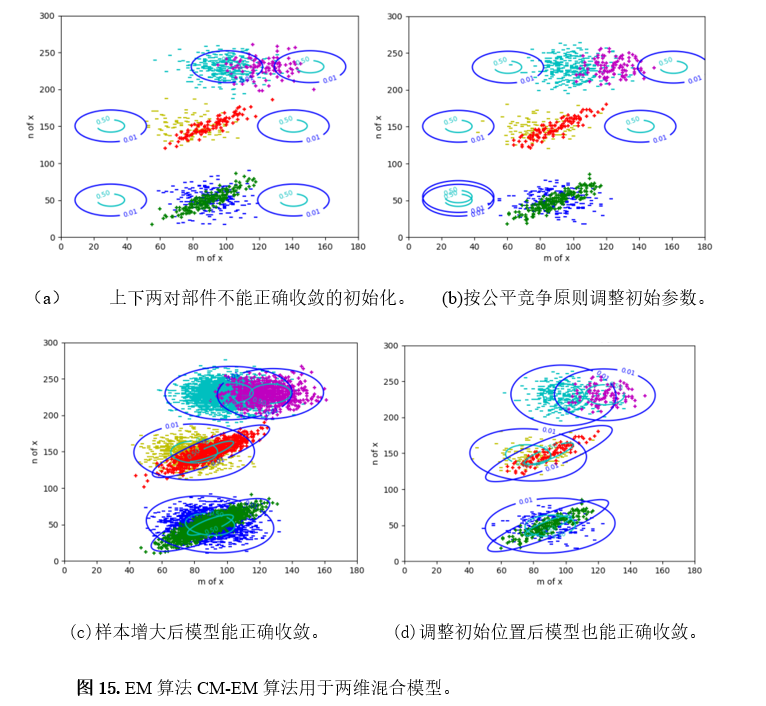2020年9月于英文期刊Information发表的一篇总结性文章

 中文: 摘要: 机器学习的一个重要问题是：在标签数目n>2时，构造和优化一组学习函数并且希望它在先验分布P(x)(x是一个实例)改变时仍然有用。 要解决这个问题是非常困难的。为解决这一问题，语义信息G理论、逻辑贝叶斯推断(Logical Bayesian Inference，缩写为LBI)和一组信道匹配(Channel Matching，缩写为CM)算法一起构成一个系统解决方案。在语义信息G理论(或G理论)中，一个语义信道由一组真值函数或隶属函数构成。和流行的方法中使用的似然函数、贝叶斯后验和Logistic函数相比，隶属函数能更方便地用作学习函数，并且能避免上述困难。使用LBI，每个标签的学习是独立的。多标签学习时，我们能从一个足够大的带标签样本直接获得一组优化的真值函数，不需要为不同的标签准备不同的样本。基于G理论和LBI，我们得到一组用于机器学习信道匹配算法。在最大互信息分类的例子中，两维空间有三个呈高斯分布的类别，对于大多数初始划分，2-3次迭代就能使三个标签和三个类别之间的互信息达到其最大值的99%。在混合模型的例子中，期望-最大(Expectation-Maxmization，缩写为EM) 算法被改进为信道匹配EM (CM-EM)算法，它对于某些容易导致局部收敛的混合模型有较好表现。G理论中的信息率逼真函数能帮助我们证明EM算法和CM-EM算法收敛。对于高维特征空间的最大互信息分类，我们还需要结合CM迭代算法和合神经网络做进一步研究。为了统一统计和逻辑，LBI也需要进一步研究。 关键词：语义信息论；贝叶斯推断；机器学习；多标签分类；最大互信息分类；混合模型； 确证测度；真值函数。 English: Semantic Information G Theory and Logical Bayesian Inference for Machine Learning Abstract:  Many researchers want to unify probability and logic by defining logical probability or probabilistic logic reasonably. This paper tries to unify statistics and logic so that we can use both statistical probability and logical probability at the same time. For this purpose, this paper proposes the P–T probability framework, which is assembled with Shannon’s statistical probability framework for communication, Kolmogorov’s probability axioms for logical probability, and Zadeh’s membership functions used as truth functions. Two kinds of probabilities are connected by an extended Bayes’ theorem, with which we can convert a likelihood function and a truth function from one to another. Hence, we can train truth functions (in logic) by sampling distributions (in statistics). This probability framework was developed in the author’s long-term studies on semantic information, statistical learning, and color vision. This paper first proposes the P–T probability framework and explains different probabilities in it by its applications to semantic information theory. Then, this framework and the semantic information methods are applied to statistical learning, statistical mechanics, hypothesis evaluation (including falsification), confirmation, and Bayesian reasoning. Theoretical applications illustrate the reasonability and practicability of this framework. This framework is helpful for interpretable AI. To interpret neural networks, we need further study. Keywords: semantic information theory; Bayesian inference; machine learning; multilabel classifications; maximum mutual information classifications; mixture models; confirmation measure; truth function.